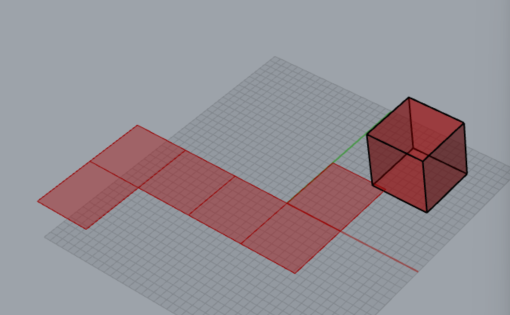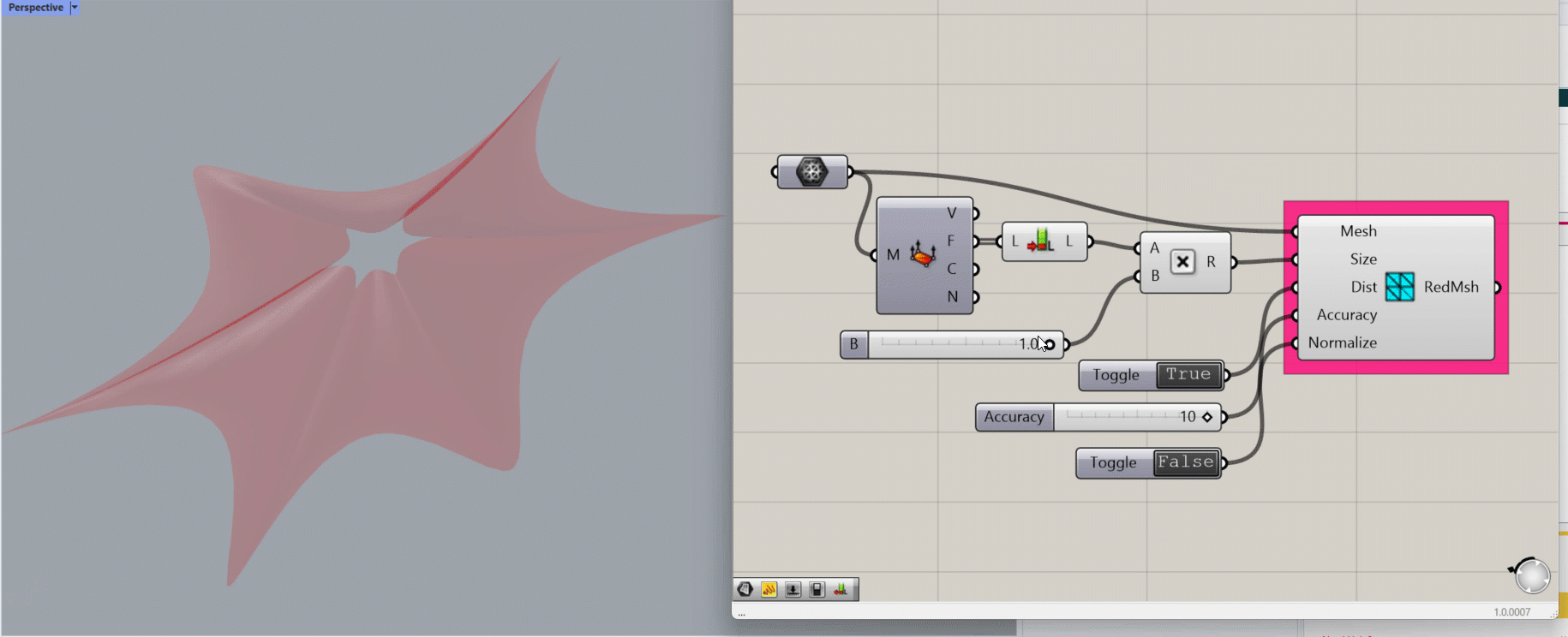# Util

LunchBox’s Util components provide a variety of utilities to users. This ‘grab bag’ of tools is designed to give useful shortcuts to Lunchbox users.

#### Component Descriptions

Below, each component is described and visual examples are given. We’ve also included special Notes and Tips that can help users with less familiar situations.

#### ArcDivide

Turns a non-uniform spline curve into a rationalized curve made up of tangential arcs.

Input:

• A list of curves to rationalize
• A list of “t” parameter values along the curve. Tip: You can use the DivideCurve parameter to determine these.

Results:

• A list of rational, planar arcs
• A list of the center points of each arc

#### Deconstruct Wireframe

Organizes a wireframes into a list of nodes and gives definition to its curves.

Input:

• A list of curves, from a wireframe

Results:

• A list of wireframe nodes
• A list of Curve Definitions, where the numbers in each branch represent the index number of the nodes at each end of each wireframe curve.

#### Flatness Check

Checks a list of panels for their flatness, plane normals, and any deviations from flatness.

Input:

• A list of panels

Results:

• A list of flat panels (adjusted from the input list)
• A list of planes that the flat panels have been projected to
• A list of numerical deviations from the original geometry

#### Patch Surface

Returns a patch using a list of edge curves. This may not necessarily result in patches that create a watertight object, depending on the geometry and tolerance given.

Input:

• A list of edge curves as closed polylines. These do not have to be planar.
• A specified U value, the surface subdivisions in one direction.
• A specified V value, the surface subdivisions in the other direction.
• A specified tolerance (the default is 0.001)

Returns a list of patches as surfaces.

#### Random Split List

Randomly splits a list into two lists

Input:

• A list
• A random Seed (a whole number)
• A percentage, indicating how much of the list to split off (into List B).

Results:

• List A (the items remaining from the original list)
• List B (the items that have been split out)

#### Rebuild Surface

Reconstructs surfaces to the specified U and V divisions.

Input:

• A list of untrimmed surfaces
• A list of whole numbers to set the uDegrees (allowable deviation in the U direction)
• A list of whole numbers to set the vDegrees (allowable deviation in the V direction)
• A list of whole numbers to set the number of U divisions on the surface(s)
• A list of whole numbers to set the number of V divisions on the surface(s)

Results: A list of rebuilt surface(s)

#### Relative Coordinates

Returns the coordinates of a point relative to a given plane.

Input:

• A plane or list of planes to use as the basis of the point coordinates
• A list of points to coordinate

Results:

• A list of points, as (X,Y,Z) coordinates
• The X values of the deconstructed points
• The Y values of the deconstructed points
• The Z values of the deconstructed points

#### Reverse Surface Direction

Reverses the U and/or V directions on a surface.

Input:

• A list of surfaces
• A whole number between 0 and 3, indicating which elements to reverse.
• 0 = Do Nothing
• 1 = Reverse U
• 2 = Reverse V
• 3 = Reverse UV

Results:

• A list of reconstructed surfaces

Note: In the example images, the surface evaluation yields different results for its Normal, U, and V values based on the Reverse Option (R) input.

#### Unroll Brep

Creates a 2D surface that represents the faces of a 3D Brep. When folded, the unrolled Brep reforms the input Brep.

Input:

• A list of Breps to unroll.

Results: A 2D arrangement of surfaces, representing the unrolled Brep.Results of the script above, where the cube is the input value and the flattened surface is the Unrolled Brep.

#### Mesh Edges (with Tolerance)

Finds Naked Edges and Edges that are adjacent to another, with an angle greater than that specified.

Input:

• A list of mesh objects
• An integer to set the angle of tolerance.

Results:

• A list of Interior Edges
• A list of Naked Edges

#### Mesh Reduce

Reduce the number of faces on a mesh, to simplify it’s geometry.

Input:

• A mesh
• The number of faces to reduce the mesh to, called Size
• A Boolean toggle to enable or disable Distortion. Setting it to true allows the mesh to distort.
• A number between 0 and 10 to determine the accuracy of the mesh rebuild.
• A Boolean toggle to enable or disable Normalization, which will attempt to make the resulting mesh faces of similar size.

Returns a mesh with the specified number of faces.Demonstrating the balance between adjusting the input on “Size” and “Accuracy”. The greater the reduction in Size, the higher the level of Accuracy needed to maintain the mesh’s original shape.

#### Sort Duplicate Breps

Sorts a list into Unique Breps and returns a list of the indices where Breps are duplicated. Breps must be defined identically to be identified (including their location).

Input:

• A list of Breps

Returns:

• A list of Unique breps
• A list of the duplicated breps

#### Sort Duplicate Curves

Sorts a list of curves based on duplicates using document tolerances.

Input:

• A list of curves to sort

Returns:

• A list of Unique curves
• A list of Indices for each unique curve

#### Sort Duplicate Points

Sort a list of points based on duplicates.

Input:

• A list of points to sort.

Returns:

• A list of unique points
• A list of the indices of each unique point.